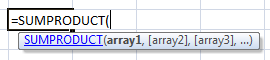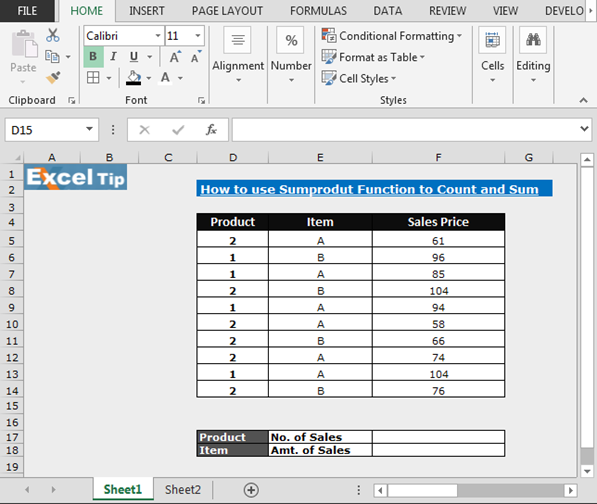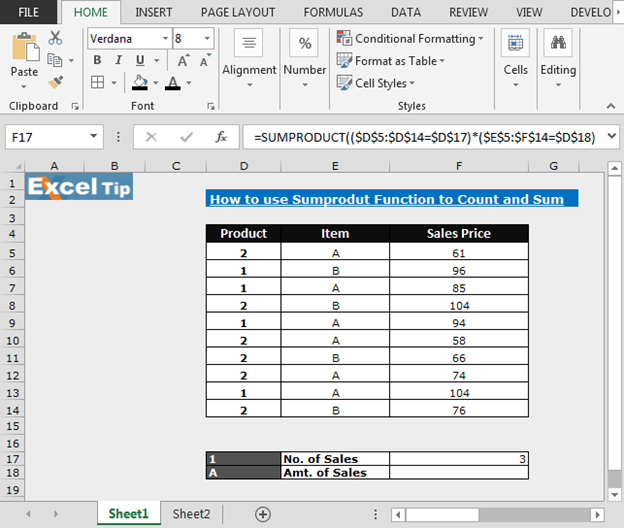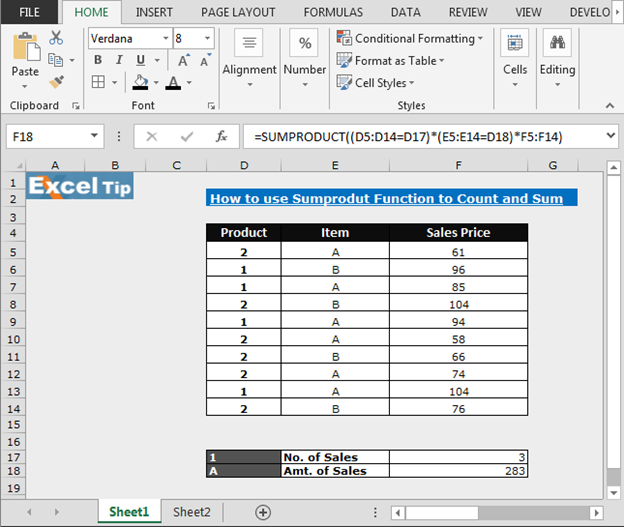# Modifying a SUMPRODUCT function to Return a Sum Rather than a Count

In this article, we are going to learn how to modify the SUMPRODUCT function to return sum rather than a count.

We use Sumproduct function to return the sum of the product of parallel ranges or arrays. Now, we use the SUMPRODUCT function to return sum.Let’s take an example and understand:-

We have data in range D4:F14. Column D contains product, Column E contains Item and column F contains sales price.

By using SUMPRODUCT, we will return the number of sales for the Product 1 and item A and then we will modify it to return the sum for the same criteria.In Cell D17, we have Product’s drop down list and in cell D18, we have Item’s drop down list. In the drop down list, when we change the product, theitem no. of sales will be changed according to the criteria.

• Enter the formula in Cell F17
• =SUMPRODUCT((\$D\$5:\$D\$14=\$D\$17)*(\$E\$5:\$F\$14=\$D\$18))
• Press Enter
• Now select the Product 1 and Item A
• Formula will return 3
• It means 3 sold quantity falls under A product and item 1To make the SUMPRODUCT formula to sum the values from a range instead of counting them, add another argument to the SUMPRODUCT formula that contains the range to sum (cells F5:F14)

To modify the Sumproduct function in order to return the Sum rather than count, follow below given steps:-

• Enter the formula in Cell F18
• =SUMPRODUCT((D5:D14=D17)*(E5:E14=D18)*F5:F14)
• Press Enter
• Now select the Product 1 and Item A
• Formula will return 283
• It means 283 quantities  under the A product and item 1 are soldIn this way, we can use Sumproduct function in place of Countif and Sumif, in Microsoft Excel.